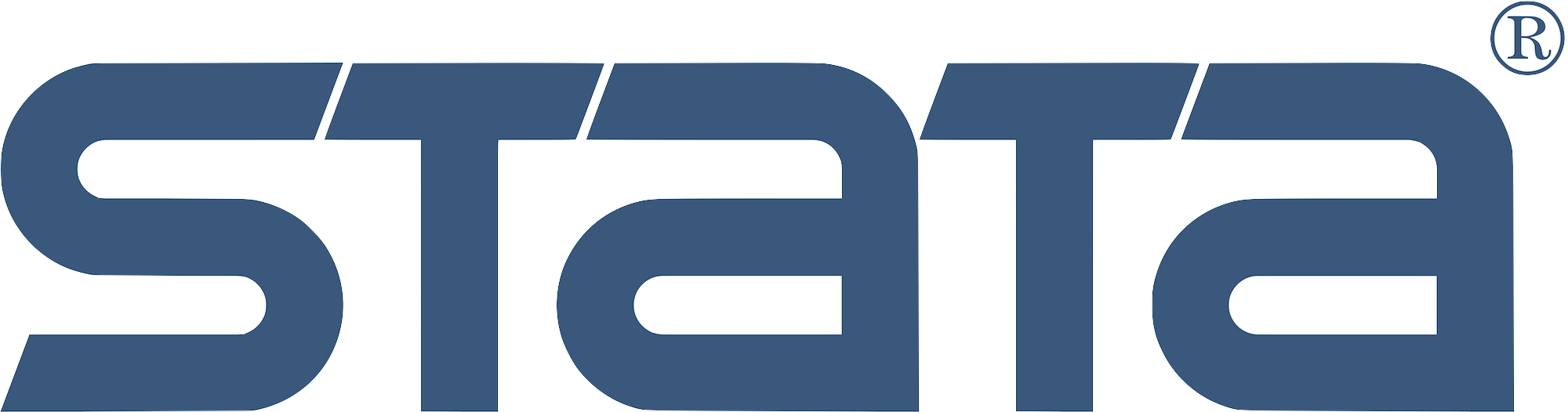# Learn Stata

### Statistical Graphics Using Stata## NetCourse®120: Statistical Graphics Using Stata

Course length: 6 weeks (4 lessons)

See dates.

## Enrol:

#### Content:

Learn how to communicate your data with Stata's powerful graphics features. This course will introduce different kinds of graphs and demonstrate how to use them for exploratory data analysis. Topics include how to use graphs to check model assumptions; how to format, save, and export your graphs for publication using the Graph Editor; how to create custom graph schemes; how to create complex graphs by layering and combining multiple graphs; how to use margins and marginsplot; and more. Bonus material includes information on user-written graph commands and useful data management tools.

#### Prerequisites:

• Stata 18 installed and working
• Basic knowledge of using Stata interactively
• Internet web browser, installed and working

Note: This course is platform independent. You can use Stata on Windows, Mac or Linux.

#### Trainer: Kerry Kammire#### Trainer: Derek Wagner#### Course content

##### Lesson 1: Getting to know your data using graphs
• Introduction
• Why graphs are an important tool for exploratory data analysis
• Data management tools for graphing data
• The example dataset
• How to create and edit basic graphs using Stata
• How to create and edit graphs with dialog boxes
• How to edit graphs with the Graph Editor
• How to create and edit graphs with commands
• Some basic graphs
• Graphs for one continuous variable
• Graphs for one categorical variable
• Graphs for two continuous variables
• Graphs for two categorical variables
• Graphs for one continuous and one categorical variable
• Graphs for many variables
• Storing, saving, and exporting graphs
• Storing graphs in memory
• Saving graphs to disk
• Exporting graphs in .png format
• Automating the process: Looping and saving
##### Lesson 2: Understanding your results using graphs
• Introduction
• Model checking using graphs
• Using the predict command
• Checking model assumptions
• Checking the normality assumption
• Checking the linearity assumption
• Checking the homoskedasticity assumption
• Identifying outliers and influential observations
• Visualizing the results of your models
• Using the margins and marginsplot commands
• A brief review of factor variables
• Categorical independent variables
• Multiple categorical independent variables
• Continuous independent variables
• Continuous and categorical independent variables
• Average response versus response at average: The atmeans option
• Contrasts of margins
• Marginal effects: Margins of derivatives of responses
• Using contour plots to visualize continuous-by-continuous interactions

Note: There is a one-week break between the posting of Lessons 2 and 3; however, course leaders are available for discussion.

##### Lesson 3: Formatting graphs for publication
• Introduction
• Formatting titles, legends, and text boxes
• Formatting titles
• Formatting legends
• Using italics, bold, superscripts, and subscripts
• Using specialty characters
• Using different fonts
• Formatting numbers
• Formatting axes, axis labels, ticks, gridlines, graph, and plot regions
• Formatting categorical axis labels
• Formatting the x and y axes
• Formatting the x- and y-axis labels
• Formatting major and minor ticks and gridlines
• Formatting the graph and plot regions
• Controlling the aspect ratio and size of graphs
• Using schemes to change the overall look of graphs
• Using built-in schemes
• Recording and saving edits in the Graph Editor
##### Lesson 4: Advanced graphs: How to layer and combine multiple graphs
• Introduction
• Layering multiple graphs with the graph twoway command
• Basic layered graphs with one y axis
• Advanced layered graphs with one y axis
• Basic layered graphs with two y axes
• Advanced layered graphs with two y axes
• Layering multiple graphs with the addplot() option
• Basic
• Creating multiple graphs with the by() option
• Combining different graphs with the graph combine command
• Basics: Making a table of separate graphs
• Advanced: Making a single complex graph from separate graphs
• Exporting graphs for publication
• Exporting graphs in pixel-based formats
• Exporting graphs in vector-based formats

Note:The previous four lessons constitute the core material of the course. The following material is optional and introduces user-written graphic commands and useful data management tools.

##### Bonus material
• Introduction
• User-written graph commands
• The Statistical Software Components (SSC) archive
• The coefplot package by Ben Jann
• How to write a simple graphics wrapper command
• How to create animated graphs
• Some fun graphs
• How to create normal curves with shaded tails
• How to show scatterplots with regression lines and residuals
• How to add normal curves to regression lines
• How to graph a histogram with a box plot
##### Appendix: Data management tools useful for graphing data
• The destring and encode commands
• The recode command
• The tabulate command with the generate() option
• The egen command
• The contract and collapse commands
• The statsby command
• The snapshot command
• The reshape command
• Macros and loops
• Extracting value labels to local macros